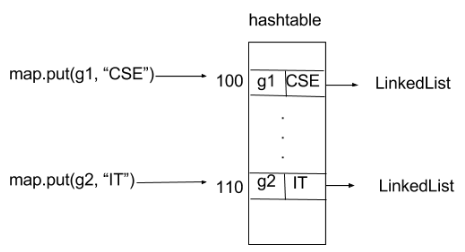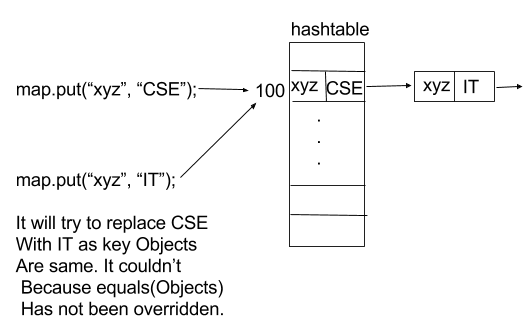Related Articles

# Why to Override equals(Object) and hashCode() method ?

• Difficulty Level : Medium
• Last Updated : 26 Sep, 2018

Prerequisite – Equals and Hashcode method
HashMap and HashSet use the hashcode value of an object to find out how the object would be stored in the collection, and subsequently hashcode is used to help locate the object in the collection. Hashing retrieval involves:

1. First, find out the right bucket using hashCode().
2. Secondly, search the bucket for the right element using equals()

Let us consider all the cases of Overriding in these methods

Attention reader! Don’t stop learning now. Get hold of all the important Java Foundation and Collections concepts with the Fundamentals of Java and Java Collections Course at a student-friendly price and become industry ready. To complete your preparation from learning a language to DS Algo and many more,  please refer Complete Interview Preparation Course.

Case 1: Overriding both equals(Object) and hashCode() method

You must override hashCode() in every class that overrides equals(). Failure to do so will result in a violation of the general contract for Object.hashCode(), which will prevent your class from functioning properly in conjunction with all hash-based collections, including HashMap, HashSet, and Hashtable. (-Joshua Bloch)
Here is the contract, from the java.lang.Object specialization:

• Whenever it(hashcode) is invoked on the same object more than once during an execution of a Java application, the hashCode method must consistently return the same integer, provided no information used in equals comparisons on the object is modified. This integer need not remain consistent from one execution of an application to another execution of the same application.
• If two objects are equal according to the equals(Object) method, then calling the hashCode method on each of the two objects must produce the same integer result.
• It is not required that if two objects are unequal according to the equals(java.lang.Object) method, then calling the hashCode method on each of the two objects must produce distinct integer results. However, the programmer should be aware that producing distinct integer results for unequal objects may improve the performance of hashtables.
 `// Java program to illustrate``// overriding of equals and``// hashcode methods``import` `java.io.*;``import` `java.util.*;`` ` `class` `Geek ``{``     ` `    ``String name;``    ``int` `id;``     ` `    ``Geek(String name, ``int` `id)``    ``{``         ` `        ``this``.name = name;``        ``this``.id = id;``    ``}``     ` `    ``@Override``    ``public` `boolean` `equals(Object obj)``    ``{``         ` `    ``// if both the object references are ``    ``// referring to the same object.``    ``if``(``this` `== obj)``            ``return` `true``;``         ` `        ``// it checks if the argument is of the ``        ``// type Geek by comparing the classes ``        ``// of the passed argument and this object.``        ``// if(!(obj instanceof Geek)) return false; ---> avoid.``        ``if``(obj == ``null` `|| obj.getClass()!= ``this``.getClass())``            ``return` `false``;``         ` `        ``// type casting of the argument. ``        ``Geek geek = (Geek) obj;``         ` `        ``// comparing the state of argument with ``        ``// the state of 'this' Object.``        ``return` `(geek.name.equals(``this``.name)  && geek.id == ``this``.id);``    ``}``     ` `    ``@Override``    ``public` `int` `hashCode()``    ``{``         ` `        ``// We are returning the Geek_id ``        ``// as a hashcode value.``        ``// we can also return some ``        ``// other calculated value or may``        ``// be memory address of the ``        ``// Object on which it is invoked. ``        ``// it depends on how you implement ``        ``// hashCode() method.``        ``return` `this``.id;``    ``}``     ` `}`` ` `// Driver code``class` `GFG ``{``    ``public` `static` `void` `main (String[] args) ``    ``{``         ` `        ``// creating two Objects with ``        ``// same state``        ``Geek g1 = ``new` `Geek(``"aditya"``, ``1``);``        ``Geek g2 = ``new` `Geek(``"aditya"``, ``1``);``         ` `        ``Map map = ``new` `HashMap();``        ``map.put(g1, ``"CSE"``);``        ``map.put(g2, ``"IT"``);``         ` `        ``for``(Geek geek : map.keySet())``        ``{``            ``System.out.println(map.get(geek).toString());``        ``}`` ` `    ``}``}`

output:

```IT
```

In this case we override both methods properly.
When we call map.put(g1, “CSE”); it will hash to some bucket location and when we call map.put(g2, “IT”);, it will generates same hashcode value (same as g1) and replace first value by second value because while iterating over same bucket it found a k such that k.equals(g2) is true, means searching key already exist. So, it replaces old value of that key by new value.

Case 2 : Overriding only the equals(Object) method

If we only override equals(Object) method, when we call map.put(g1, “CSE”); it will hash to some bucket location and when we call map.put(g2, “IT”); it will hash to some other bucket location because of different hashcode value as hashCode() method has not been overridden.As you can see clearly in image, both values are getting stored into different bucket locations. Like that every insert into map will get different bucket location whether we are using same key objects or different i.e. state of key objects is same or different.

 `// Java program to illustrate``// Overriding only the equals(Object) method``import` `java.io.*;``import` `java.util.*;`` ` `class` `Geek ``{``    ``String name;``    ``int` `id;``      ` `    ``Geek(String name, ``int` `id)``    ``{``        ``this``.name = name;``        ``this``.id = id;``     ``}``      ` `    ``@Override``    ``public` `boolean` `equals(Object obj)``    ``{``       ``// if both the object references are ``       ``// referring to the same object.``       ``if``(``this` `== obj)``            ``return` `true``;``           ` `        ``// it checks if the argument is of the ``        ``// type Geek by comparing the classes ``        ``// of the passed argument and this object.``        ``// if(!(obj instanceof Geek)) return false; ---> avoid.``        ``if``(obj == ``null` `|| obj.getClass()!= ``this``.getClass())``            ``return` `false``;``           ` `        ``// type casting of the argument.    ``        ``Geek geek = (Geek) obj;``           ` `        ``// comparing the state of argument with ``        ``// the state of 'this' Object.``        ``return` `(geek.name.equals(``this``.name) && geek.id == ``this``.id);``    ``}``}`` ` `class` `GFG ``{``    ``public` `static` `void` `main (String[] args) ``    ``{``         ` `        ``// creating two Objects with ``        ``// same state``        ``Geek g1 = ``new` `Geek(``"aditya"``, ``1``);``        ``Geek g2 = ``new` `Geek(``"aditya"``, ``1``);``         ` `        ``Map map = ``new` `HashMap();``        ``map.put(g1, ``"CSE"``);``        ``map.put(g2, ``"IT"``);``         ` `        ``for``(Geek geek : map.keySet())``        ``{``            ``System.out.println(map.get(geek).toString());``        ``}`` ` `    ``}``}`

Output:

```CSE
IT```

Case 3: Overriding only hashCode() method

Consider another example of map :

```Map map = new HashMap();
map.put(“xyz”, “CSE”);
map.put(“xyz”, “IT”);
```

When we call map.put(“xyz”, “CSE”); it will generate hashcode value and stores it to the bucket location that is specified with this address (hashcode value). And when we call map.put(“xyz”, “IT”); it generates same hashcode value as previous entry since key objects are same and hashCode() method has been overridden. So it should replace first with second as per rule. But it didn’t. Reason is, when it iterate through that bucket and seeks to find k such that k.equals(“xyz”) i.e. if searching key already exist. But it fails to find because equals(Object ) method has not been overridden. It is violation of rule of hashing.

 `// Java program to illustrate ``// Overriding only hashCode() method`` ` `import` `java.io.*;``import` `java.util.*;`` ` `class` `Geek ``{``    ``String name;``    ``int` `id;``      ` `    ``Geek(String name, ``int` `id)``    ``{``        ``this``.name = name;``        ``this``.id = id;``     ``}``     ` `    ``@Override``    ``public` `int` `hashCode()``    ``{``          ` `        ``// We are returning the Geek_id ``        ``// as a hashcode value.``        ``// we can also return some ``        ``// other calculated value or may``        ``// be memory address of the ``        ``// Object on which it is invoked. ``        ``// it depends on how you implement ``        ``// hashCode() method.``        ``return` `this``.id;``    ``}``      ` `}`` ` `class` `GFG ``{``    ``public` `static` `void` `main (String[] args)``    ``{``         ` `        ``// creating two Objects with ``        ``// same state``        ``Geek g1 = ``new` `Geek(``"aditya"``, ``1``);``        ``Geek g2 = ``new` `Geek(``"aditya"``, ``1``);``         ` `        ``Map map = ``new` `HashMap();``        ``map.put(g1, ``"CSE"``);``        ``map.put(g2, ``"IT"``);``         ` `        ``for``(Geek geek : map.keySet())``        ``{``            ``System.out.println(map.get(geek).toString());``        ``}`` ` `    ``}``}`

Output:

```CSE
IT```In above diagram when we call map.put(“xyz”, “IT”); then it tried to replace first value (CSE) by second value(IT) but it was not possible so it insert second pair (key, value) into a new LinkedList node that hashmap internally use. It it total violation of rule as key are unique in map.

Reference : StackOverflow

This article is contributed by Nitsdheerendra. If you like GeeksforGeeks and would like to contribute, you can also write an article using contribute.geeksforgeeks.org or mail your article to contribute@geeksforgeeks.org. See your article appearing on the GeeksforGeeks main page and help other Geeks.